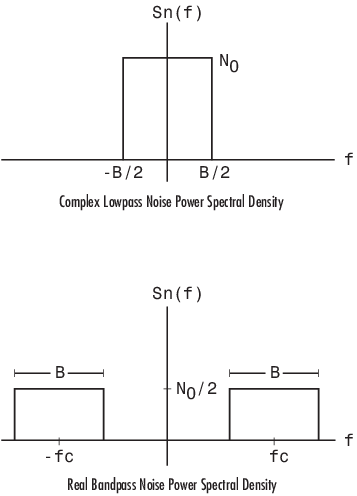## AWGN Channel

### Section Overview

An AWGN channel adds white Gaussian noise to the signal that passes through it. You can create an AWGN channel in a model using the `comm.AWGNChannel` System object™, the AWGN Channel block, or the `awgn` function.

The following examples use an AWGN Channel: QPSK Transmitter and Receiver and General QAM Modulation in AWGN Channel.

### AWGN Channel Noise Level

Typical quantities used to describe the relative power of noise in an AWGN channel include

• Signal-to-noise ratio (SNR) per sample. SNR is the actual input parameter to the `awgn` function.

• Ratio of bit energy to noise power spectral density (EbN0). This quantity is used by `BER Analyzer` Tool and performance evaluation functions in this toolbox.

• Ratio of symbol energy to noise power spectral density (EsN0)

#### Relationship Between EsN0 and EbN0

The relationship between EsN0 and EbN0, both expressed in dB, is as follows:

where k is the number of information bits per symbol.

In a communications system, k might be influenced by the size of the modulation alphabet or the code rate of an error-control code. For example, in a system using a rate-1/2 code and 8-PSK modulation, the number of information bits per symbol (k) is the product of the code rate and the number of coded bits per modulated symbol. Specifically, (1/2) log2(8) = 3/2. In such a system, three information bits correspond to six coded bits, which in turn correspond to two 8-PSK symbols.

#### Relationship Between EsN0 and SNR

The relationship between EsN0 and SNR, both expressed in dB, is as follows:

where Tsym is the symbol period of the signal and Tsamp is the sampling period of the signal.

For a complex baseband signal oversampled by a factor of 4, the EsN0 exceeds the corresponding SNR by 10 log10(4).

Derivation for Complex Input Signals.  You can derive the relationship between EsN0 and SNR for complex input signals as follows:

where

• S = Input signal power, in watts

• N = Noise power, in watts

• Bn = Noise bandwidth, in Hertz = Fs = 1/Tsamp.

• Fs = Sampling frequency, in Hertz

Behavior for Real and Complex Input Signals.  These figures illustrate the difference between the real and complex cases by showing the noise power spectral densities of a real bandpass white noise process and its complex lowpass equivalent.